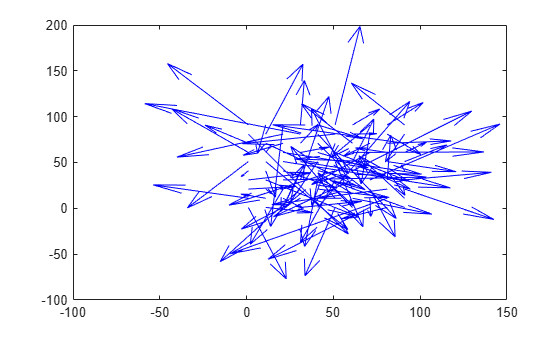opticalFlow

Object for storing optical flow matrices

Description

The opticalFlow object stores the direction and speed of a moving object from one image or video frame to another. Use the object function plot to plot the optical flow vectors.

Creation

Description

example

flow = opticalFlow creates an optical flow object with default property values.

example

flow = opticalFlow(Vx,Vy) creates an optical flow object from two equal-sized matrices Vx and Vy. The matrices Vx and Vy are the x and y components of velocity respectively. The input velocity components set the Properties of optical flow object.

Input Arguments

expand all

x component of velocity, specified as an M-by-N matrix.

Data Types: single | double

y component of velocity, specified as an M-by-N matrix.

Data Types: single | double

Note

Vx and Vy must be of the same size and data type.

Properties

expand all

x component of velocity, specified as an M-by-N matrix. If the input Vx is not specified, the default value of this property is set to 0-by-1 empty matrix.

Data Types: single | double

y component of velocity, specified as an M-by-N matrix. If the input Vy is not specified, the default value of this property is set to 0-by-1 empty matrix.

Data Types: single | double

Phase angles of optical flow in radians, specified as an M-by-N matrix of the same size and data type as the components of velocity. The phase angles of optical flow is calculated from the x and y components of velocity. If the inputs Vx and Vy are not specified, the default value of this property is set to 0-by-1 empty matrix.

Data Types: single | double

Magnitude of optical flow, specified as an M-by-N matrix of the same size and data type as the components of velocity. The magnitude of optical flow is calculated from the x and y components of velocity. If the inputs Vx and Vy are not specified, the default value of this property is set to 0-by-1 empty matrix.

Data Types: single | double

Object Functions

 plot Plot optical flow vectors

Examples

collapse all

Create an optical flow object and view its properties.

flow = opticalFlow
flow =

opticalFlow with properties:

Vx: [0×1 double]
Vy: [0×1 double]
Orientation: [0×1 double]
Magnitude: [0×1 double]

The default values of the properties are returned as an empty matrix of size 0-by-1 and data type double.

Create an optical flow object from two equal-sized velocity matrices.

Vx = randn(100,100);
Vy = randn(100,100);
opflow = opticalFlow(Vx,Vy);

Inspect the properties of the optical flow object. The orientation and the magnitude are computed from the velocity matrices.

opflow
opflow =
opticalFlow with properties:

Vx: [100x100 double]
Vy: [100x100 double]
Orientation: [100x100 double]
Magnitude: [100x100 double]

Plot the velocity of the object as a quiver plot.

plot(opflow,'DecimationFactor',[10 10],'ScaleFactor',10);